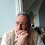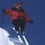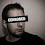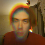## Thursday, March 11, 2010

### Theorems that you simply don't believe

There are some theorems that are surprising. I've already blogged on that (I can't seem to find the link). However, there are some theorems that some people simply do not believe. I mean people who understand the proofs and still don't believe them. Let me give you a contrast- I DO believe that NSPACE(n) is closed under complementation because, while surprising, the proof really does tell you why its true. For the following surprising results the proof does not help. Or at least does not help the people who were surprised by it.
1. Barrington's theorem. I've read it, talked to Barrington about it, and even taught it. I still don't believe that (say) the set of strings that have the number of 1's equivalent to 0 mod 101 can be done by a width 5 branching program.
2. Banach Tarski Paradox A CS grad students who knows some math says that it shows that mathematics is broken. I would prefer to say it casts doubt on the axiom of choice.
3. The classification of finite simple groups. Does any one person even know the proof? Couldn't they have missed some group? Counter argument: the list is on Wikipedia so it has to be correct.
4. The rationals and naturals are the same size. I know someone who knows the proof and is happy to say they are the same cardinality but refuses to say they are the same size. (I think they are wrong and this is important- using the term size DOES matter.)
5. A well known theorist told me that he used to believe both P ≠ BPP and there were problems in DTIME(2O(n)) that require circuits of size 2&Omega(n);. Oh well.
6. Lance Fortnow tells me he has a hard time believing the Recursion Theorem. Perhaps because the proof is completely uninformative. (Ted Slaman, a well known recursion theorists, agrees that the proof is uninformative. Bob Soare thinks the proof is quite intuitive- a failed diag argument.)
7. Probability has a few of these: The Central Limit Theorem says that stuff is all normal. That can't be true! I've done the calculations for Birthday Paradox but it still seems suspect to me. And don't get me started on The Monty Hall Paradox.
8. Local Lovasz Lemma has gone from being something I didn't believe to something I now understand and believe. The original proof just looked like symbols being pushed around, but Moser's and later Moser-Tardos's constructive versions makes sense to me.
9. We all know that Godel's theorem surprised people- but were there people who did not believe it? This theorem does not surprise Generation Xers who are not at all surprised to find out certain problems cannot be solved. Their response: Whatever.
10. The existence of Geometries that are as valid as Euclidean but not Euclidean. Again, this surprised people, but were there those who did not believe it? In this age of moral relativism people have no problem with different geometries that are all valid.
How about you? Are there any theorems that you simply don't believe?

1.I had a geometry teacher in high school who refused to believe in non-Euclidean geometries.

2.I had the hardest time convincing one young man that 1=0.9999... All three proofs I showed him he believed, but thought it was probably a joke.

That I showed him the division by zero "proof" that 1=2 the week before probably didn't help.

3.I think with some proofs it is just a matter of finding the right explanation that makes sense to you. I would put Monty Hall, Birthday Paradox, Lovasz Local Lemma in this category.

But other proofs are really more philosophical, they define things in ways that we are not used to or expose issues with the underlying model. I would put Cantor, Godel and Banach-Tarski in this category.

4.How does one not believe a theorem? It's simply a consequence of some premises.

I may hold that a theorem is vacuously true, in the sense that it has no interpretation in which the premises are true, but that is a different matter.

For instance, if the Godel Theorem you refer to is the one which constructs an arithmetical formula [R(x)] such that neither [(Ax)R(x)] nor [~(Ax)R(x)] is provable from the PA axioms IF PA is omega-consistent, Godel's reasoning is flawless.

However, it is easy to show that PA is omega-consistent if, and only if, Aristotle's Particularisation holds over the natural numbers.

(AP is the postulation that we can always conclude that:

(i) there is a natural number n such that F(n) is true,

from the assertion that:

(ii) it is not true that, for any given natural number n, F(n) is false.)

Now, I may argue that - as Brouwer steadfastly held - AP does not hold over the natural numbers.

In fact, such an argument follows rather straightforwardly from the first half of the proof of Godel's Theorem, where he shows that [(Ax)R(x)] is not PA-provable even though, for any given numeral [n], [R(n)] is PA-provable.

Hence PA is not omega-consistent.

So, even though I may not accept that Godel's Theorem has shown that there are certain problems which cannot be solved, I can only argue that the Theorem is vacuously true!

5.I'm surprised you left off the PCP Theorem . . .

6.Blum's Speedup Theorem definitely falls into this category.

7.Recursion theorem is one of my favorite ones. I would suggest to take a look at Kleene's work, after they got a paradox out of Church's original lambda calculus, he just translated it to general recursive functions and we got the recursion theorem. I prefer the fix point version though. An easy way to remember the proof is the following:

1. This sentence is false.

2. If you write the following expression inside quotes twice, the second time inside quotes, the resulting sentence is false: "
If you write the following expression inside quotes twice, the second time inside quotes, the resulting sentence is false: "

8.I don't believe in math, I just do it.

9.For me Monty Hall is as easy as it gets.

10.I can't believe that you can't believe the lovasz local lemma or the monty hall problem. These theorems are very intuitive, though I concede that the literature does not explain them properly. In general, I find that mathematicians, unlike computer scientists, often do not "understand" the things that they prove and are not bothered by it. Therefore, since they operate with the working assumption that symbol manipulation is a perfectly valid way of thinking about a problem, they present the proof in a completely unintuitive way. Let me illustrate via the lovasz local lemma and the monty hall problem:

1- LLL: If you look at the symmetric LLL, it simply is very unintuitive. The way to understand it is to look at the asymmetric LLL. Examine the proof as in the Alon Spencer book, and consider the following reinterpretation: Since the dependencies between the events are few, you are simply bounding the "blowup" in conditional probability of a bad event! Once you start thinking of it this way, it becomes obvious!

2- The Monty hall problem: Instead of 3 doors, consider what happens with a million doors and a game show host that reveals all but two doors. Then it becomes obvious. If its true with a million, it must be true with 3 though less obvious.

11.The PCP theorem seems also quite hard to believe.

And Skolem's paradox (set theory has a countable model) is somewhat disturbing and fascinating.

12.Lots of examples in crypto. One-way functions in NC^0, and that you can do anything non-trivial (let alone all of NP) in zero knowledge...

13.To quote John von Neumann: “In mathematics you don't understand things. You just get used to them.”

What does it even mean to "believe" a theorem? Sure, lots of theorems are counter-intuitive, but why should I (or anyone else) trust my intuition more than a cold hard proof?

Now, if you meant "Are there theorems which you do not believe have really been proved?", the only one I'd list of the classification of finite simple groups. Even the provers will admit that there is no complete, self-contained, written proof. Only 10000+ pages of boring technical details remain to be checked, but we're quite sure they'll all work out.

14.Banach Tarski Paradox A CS grad students who knows some math says that it shows that mathematics is broken. I would prefer to say it casts doubt on the axiom of choice.

The point is that the pieces involved aren't at all measurable. Your ideas of what can be done geometrically really only apply to measurable pieces.

15.In physics there are tons of people who do not believe "Bell's theorem." :) Probably not what you're looking for, heh.

16.Anonymous #11: Thanks, that was really helpful for me.

17.And Skolem's paradox (set theory has a countable model) is somewhat disturbing and fascinating.

If you study set theory, you get used to this sort of thing quickly. The real lesson is that countable is a pretty shifty concept. Some objects are countable in concrete ways (say the natural numbers). But there's a lot of stuff that's countable but in far less understandable ways.

And with forcing, you can make anything set countable. Although it might no longer be the set you're interested in.

18.One reaction to Banach-Tarski could be to throw out the Axiom of Choice. But the pathology introduced by the use of choice results only because standard notions of measure are point-based. So perhaps a more reasonable solution is to consider point-free models of space and develop an appropriate notion of measure for such models (typically referred to as "gunk".)

19.You write a blog called "Computational Complexity" and doubt the solution to the Monty Hall problem? It takes about 5 minutes to code up and observe the solution in practice. Looks like simple laziness to me.

20.I still don't believe that (say) the set of strings that have the number of 1's equivalent to 0 mod 101 can be done by a width 5 branching program.

Is there any particular reason you picked 101 rather than a smaller number like 3?

I don't see what is not to believe here -- the issue is just that the size of the BP might be incredibly large. It shouldn't be too difficult to get some rough estimate of the size required, though...

21.Anonymous and bishnu, please READ what Bill wrote. He is not saying that HE doesn't believe these. He is saying he knows OTHER PEOPLE who don't "believe" them.

22.And Skolem's paradox (set theory has a countable model) is somewhat disturbing and fascinating.

Less disturbing and more fascinating once you read the 1922 address, Some remarks on axiomatized set theory", delivered in Helsinki before the Fifth Congress of Scandinavian Mathematicians, 4-7 August 1922 (reproduced in Heijenoort's 'From Frege to Godel'), in which Skolem improved upon both the argument and statement of Lowenheim's 1915 theorem---subsequently labelled as the (downwards) Lowenheim-Skolem Theorem. He then drew attention to a :

... peculiar and apparently paradoxical state of affairs. By virtue of the axioms we can prove the existence of higher cardinalities, of higher number classes, and so forth. How can it be, then, that the entire domain B can already be enumerated by means of the finite positive integers? The explanation is not difficult to find. In the axiomatization, set' does not mean an arbitrarily defined collection; the sets are nothing but objects that are connected with one another through certain relations expressed by the axioms. Hence there is no contradiction at all if a set M of the domain B is non-denumerable in the sense of the axiomatization; for this means merely that within B there occurs no one-to-one mapping Phi of M onto Z_0 (Zermelo's number sequence). Nevertheless there exists the possibility of numbering all objects in B, and therefore also the elements of M, by means of the positive integers; of course such an enumeration too is a collection of certain pairs, but this collection is not a set' (that is, it does not occur in the domain B)."

Banach Tarski Paradox A CS grad students who knows some math says that it shows that mathematics is broken. I would prefer to say it casts doubt on the axiom of choice.

As to the Axion of Choice, in the same address Skolem emphasised that the Axiom is an essentially non-verifiable statement which does not express any definable content; therefore it cannot be expected to communicate any meaningful information unambiguously under interpretation:

So long as we are on purely axiomatic ground there is, of course, nothing special to be remarked concerning the principle of choice (though, as a matter of fact, new sets are not generated univocally by applications of this axiom); but if many mathematicians---indeed, I believe, most of them---do not want to accept the principle of choice, it is because they do not have an axiomatic conception of set theory at all. They think of sets as given by specification of arbitrary collections; but then they also demand that every set be definable. We can, after all, ask: What does it mean for a set to exist if it can perhaps never be defined? It seems clear that this existence can be only a manner of speaking, which can lead only to purely formal propositions---perhaps made up of very beautiful words---about objects called sets. But most mathematicians want mathematics to deal, ultimately, with performable computing operations and not to consist of formal propositions about objects called this or that."

Skolem's remarks seem to be directed against viewing the formal consistency of a formal language as adequate for using it as a language of, both, precise expression and effective communication.

Skolem appears to caution (an advisory needed even more today) that such an approach encourages viewing mathematics as a meaningless game" by delinking the development of a formal language, first, from its roots as a formalisation of abstractions that we intend it to express precisely; and, second, from its goal as a means of communicating such abstractions effectively and unambiguously.

23.What in the world does this have to do with "belief"? Can't people either know or not know and stop pretending that 'beleving' somehow makes things less suspect?

Belief is the pretense of knowing.

24.1) PCP: Actually I DO find the proof to tell me why its true so I do believe it.

2) Coding up Monty Hall is NOT the way to understand why its true.

3) BP of width 5 for the MOD 3 would be EASY--- just keep track of the number of 1's mod 3. Mod a really large number is more puzzling for me.
The fact that the program length is long but poly length --- I don't believe it!

4) I find the following comment idiotic, though not for the reasons you might think:

he runs a complexity blog yet does not understand Monty Hall Paradox''

YES I was quoting other people.
I happen to understand the MH paradox. but that's not the point.

The KEY to knowledge is to NOT be shy about what you don't understand and ask for help on it.
People who are shy about what they don't understand and who don't seek help on it as a result remain ignorant.

ALSO- once someone understands something its hard to imagine not understanding it. This leads to a
certain kind of arrogance which the
commenter exhibited.

bill g.

bill g.

25.I simply do not believe that 25 divided by 5 is 14.
Here is the proof.

26.Lance Fortnow tells me he has a hard time believing the Recursion Theorem.

With reason!

Given a set X, an element k of X and a function f: X -> X, the theorem states that there is a unique function F: N -> X (where N denotes the set of natural numbers including zero) such that:

F(0) = k
F(n + 1) = f(F(n))

for any natural number n.

In set theory, this unique' function F is the set {{F(0), k}, {F(1), f(k)}, {F(2), f(f(k))}, ...}.

Now, it follows from Godel's Theorem VII of his seminal 1931 paper on undecidable arithmetical propositions that, given a recursive function, say f(x), we can always create an arithmetical relation r(x, y)] such that, for any given natural numbers m, n:

f(m) = n if, and only if, r(m, n) holds.

Now it can be shown that, although there is a Turing Machine such that, given any natural numbers m and n, TM will decide f(m) = n as true or false, there is no TM such that, given any natural numbers m and n, TM will decide r(m, n) as true.

(This is reflected by the assertion that the recursive function f(x) is not strongly representable in Peano Arithmetic PA.)

In other words, since f(x) = y is recursively decidable, whilst r(m, n) is not, they are instantiationally equivalent, but computationally different, number-theoretic relations.

However, by the Recursion Theorem, they both define a unique' set F in ZF, and are set-theoretically identical to it if we take k as f(0)!

This may not matter much to a set-theorist who takes comfort from Bertrand Russell's quip that mathematics is that subject where we never know what we are talking about, nor whether it is true; it should to a computationalist!

27.Probably the first theorem I ever encountered that I did not believe is the Well-Ordering theorem, esp. as it applies to the Reals. I think that was actually the first consequence of the Axiom of Choice that got folks suspicious about it.

28.Goodstein's theorem definitely falls into this category for me. The statement of the theorem is not counter-intuitive to me, because I don't have any intuition one way or the other about whether Goodstein sequences should converge. But the proof, on the other hand, is something I just cannot wrap my head around.

29.The Central Limit Theorem does not say "stuff is all normal"! As you say, that can't be true. The CLT applies to random variables with finite variance. Otherwise stable distributions are a better bet.

By requiring finite variance, one is making the assumption that really horrible outliers are not going to dominate the system's overall behaviour. That's quite an assumption.

30.Andras- THANKS for the clarification.
I will look at the Central Limit Theorem more carefully and may come to believe it
(less likely with Banach-Tarski!)

bill g.

31.Bill -- your intuition that a width-5 BP cannot do the mod-101 in poly-size probably tells you that it cannot do the mod-101 function at all. You might want to look at the proof that a width-3 permutation BP can do any function at all, including mod-101. The jump from that to the width-5 result is just quantitative...

32.Dave Barrington- THANKS, I will look at that. Are you related to the Dave Barrington that did Barrington's theorem in the first place :-)

33.I found the discussion following this post very interesting and enlightening. I can't think of which is my favorite theorem that I don't believe, but I'm surprised to see that disbelief of theorems comes as a surprise to some readers. Just knowing there's a proof out there, or even reading it, cannot be enough to really own a theorem.

Unless you are of the Russian school persuasion, where any known result is trivial by definition (just go and read the proof) and anything that hasn't been proved yet is hard by definition.

As for finite simple groups, I think we can relax "believing the proof" to: there might be a couple of sporadic groups still out there that were missed on the first pass, but there is no major flaw in any of the arguments, and in particular, no *infinite* family was overlooked. (Disclaimer: This is just a suggestion: I do not know if this accurately represents the opinion of anyone who has a right to an opinion about finite simple groups.)

34.Goodstein's theorem definitely falls into this category for me.

Yes, Goodstein's Theorem does meet the criteria of a Theorem that begs for belief. It is a striking case of proving a Theorem in ZF, and postulating that it must hold over the natural numbers! See here.

35.The Isolating Lemma of Mulmuley, Vazirani and Vazirani, which makes min wt set in *any* set system unique, by assigning poyn large random wts to elements, even though the set system may have exponentially many sets. Has found many appls.

36.The Banach-Tarski theorem is a theorem, not a paradox. Regarding that theorem, I'd say it doesn't mean math is broken, nor that it casts doubt on the axiom of choice. It does, certainly, if you're trying to model that thing we call "real world", but mathematicians stopped being concerned for that a long time ago.

37.Hi Bhup,

I think Lance meant the recursion theorem in classical recursion theory (computability theory), not the definability of a function by recursion, i.e.:

Let denotes the code of (Turing-)computable function G.
For every computable function F(x,y), there is a computable function G(x) s.t. G(x)=F(x,). Moreover code of G is computable from code of F.

IMO, recursion theorem is completely believable, as it gives you exactly how to construct the fix point. The only problem is that the proof is seldom made clear enough for the reader.

ps: are you a category theorist?

38.Sorry for resubmitting, Blogger ate my less than signs in the previous one, I replaced the code of G with 'G'.
Let 'G' denotes the code of (Turing-)computable function G.
For every computable function F(x,y), there is a computable function G(x) s.t. G(x)=F(x,'G'). Moreover code of G is computable from code of F.

39.Natural and rational numbers, and SIZE x CARDINALITY:

I think the point here is that CARDINALITY is taken as a technical term, where only the pure collection of elements is important, while SIZE is taken in the intuitive sense we often apply to structures.

Thus, preserving the usual order structures of natural and rational numbers, it is intuitively clear that the SIZE of the structure of rational numbers is GREATER than the SIZE of the structure of the natural numbers (because the latter STRUCTURE can be embedded in the former, and still there are lots of spaces left free...).

40.I think this point (size x cardinality) illustrates a point about the disbelief in theorems: maybe it is the case that mathematics is as hermeneutic as philosophy...

41.This point (size x cardinality) illuminates a curious issue about the disbeliefs in theorems: maybe it is the case that mathematics is as hermeneutic as philosophy...

42.I don't understand the point about rationals being countable. If your problem is that the word size is not the right word to use it has no relation to believability of a theorem.

43.I was really surprised recently to learn that Erdős didn't believe the Monty Hall Problem. It doesn't take too much I don't think, to look at Monty Hall and the Birthday paradox from the right angle to make sense of the conclusions.

44.Goodstein's theorem isn't that surprising if you ignore the technical nonsense about infinite ordinals. It's just structural induction on trees, a non-arithmetic concept, but completely intuitive as finite combinatorics. Only if you want to shoehorn it into the framework of arithmetic do you have to mess with ordinals. Specifically you represent trees as ordinals up to epsilon-0, which happens to be the upper limit of Peano arithmetic. But it's easier to just reason about the trees directly even though the induction principle on them is in some sense "second order".

45.Here's a paradox which tends to surprise people. It sounds deep and mysterious, but it's really just a simple countability argument: you can't prove, for every real number, that that number equals itself. (Cuz there are more real numbers than there are proofs) See: A Provability Paradox